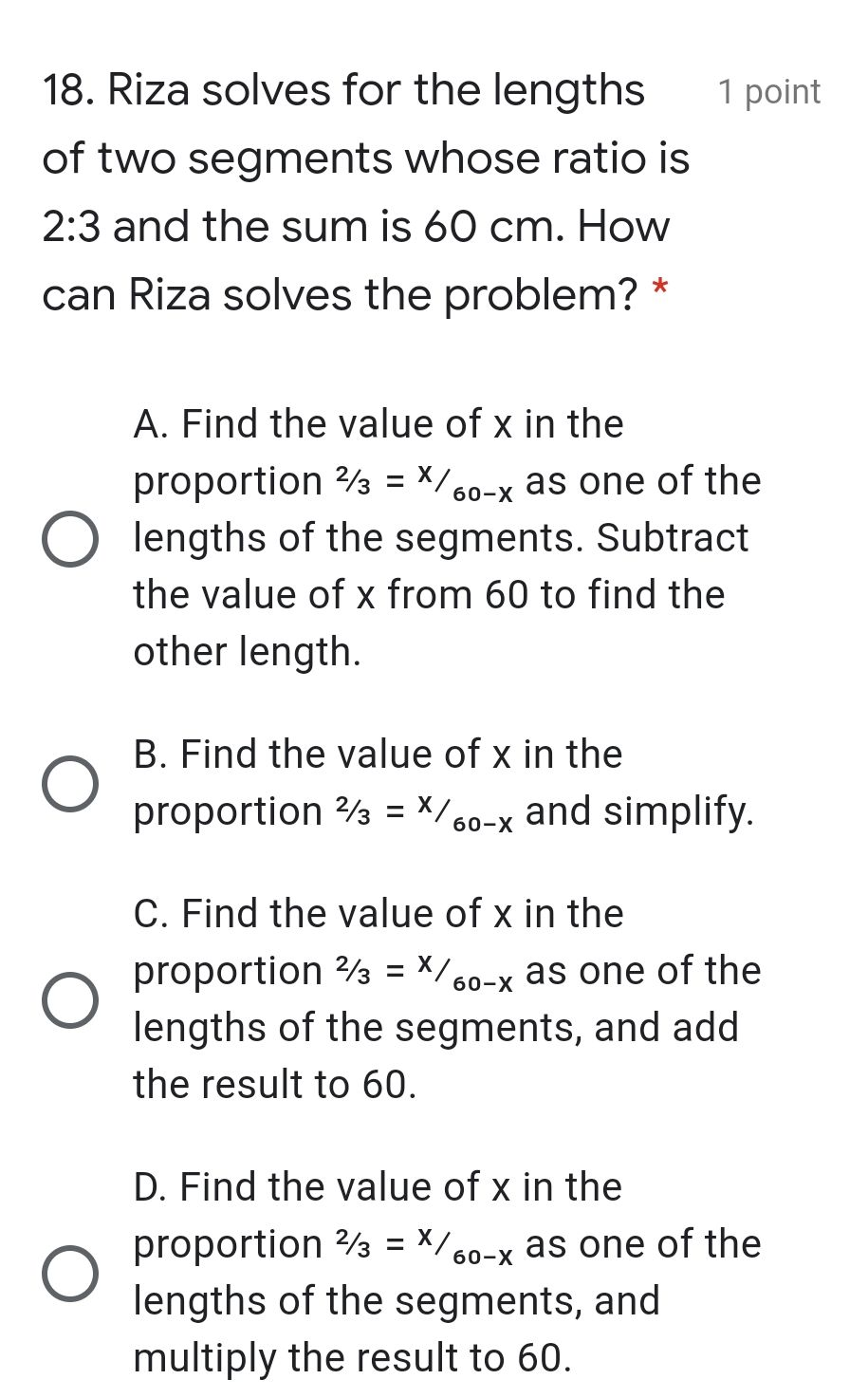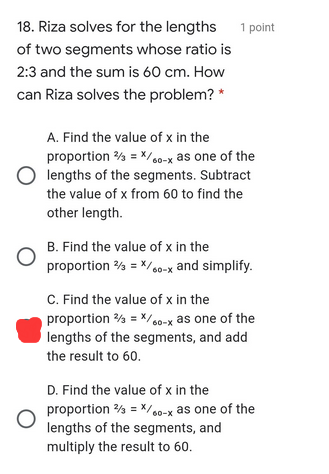### ¿Todavía tienes preguntas de matemáticas?

Pregunte a nuestros tutores expertos
Algebra
Pregunta18. Riza solves for the lengths of two segments whose ratio is $$2 : 3$$ and the sum is $$60 cm$$ . How can Riza solves the problem? $${ } ^ { * }$$

A. Find the value of $$x$$ in the proportion $$2 / 3 = x / 60 - x$$ as one of the lengths of the segments. Subtract the value of $$x$$ from $$60$$ to find the other length.

B. Find the value of $$x$$ in the proportion $$2 / 3 = x / 60 - x$$ and simplify.

C. Find the value of $$x$$ in the proportion $$2 / 3 = x / 60 - x$$ as one of the lengths of the segments, and add the result to $$60$$ .

D. Find the value of $$x$$ in the proportion $$2 / 3 = x / 60 - x$$ as one of the lengths of the segments, and multiply the result to $$60$$ .